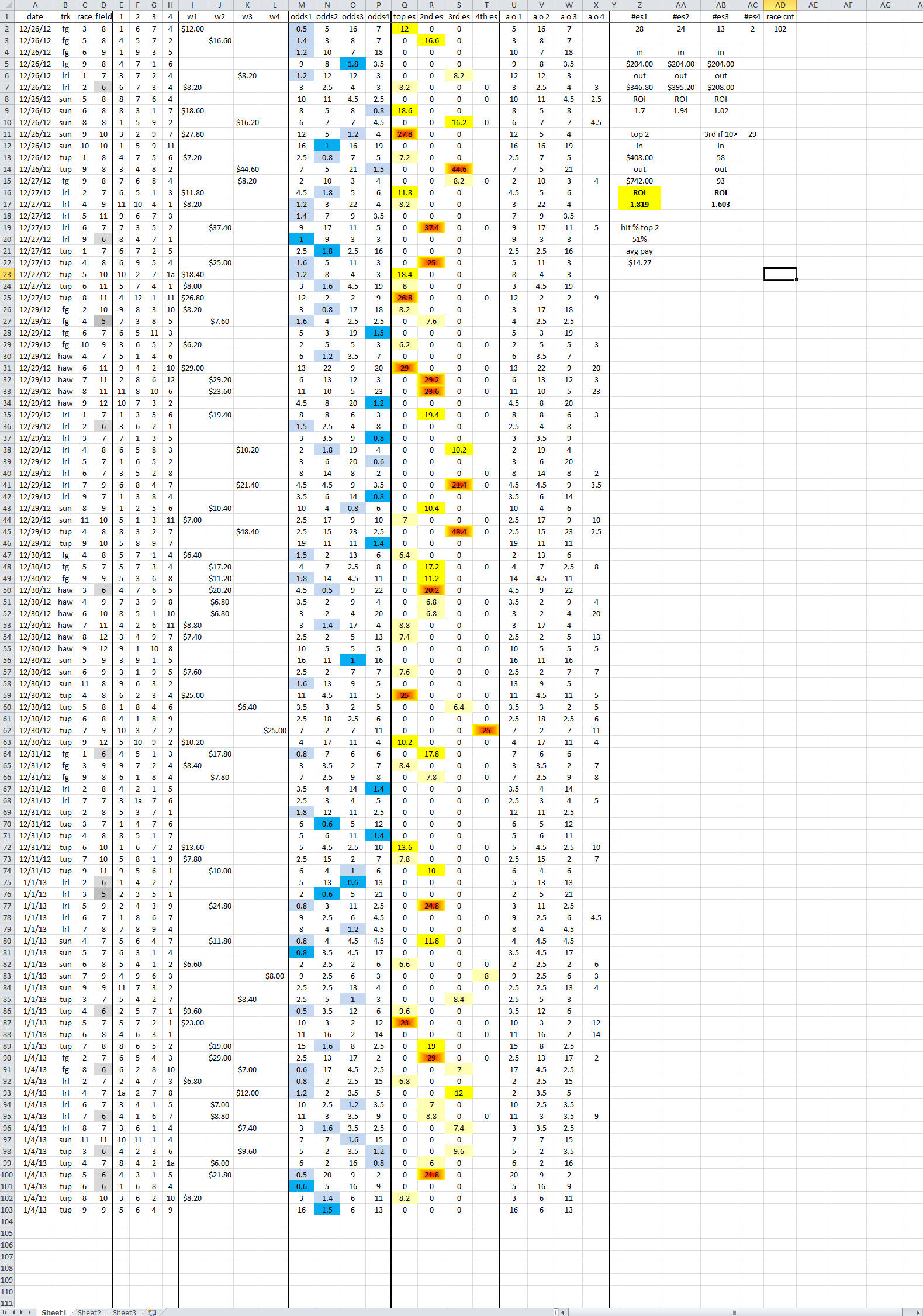# Speed 2013 Results

Explanation of the table below (use your scroll bars to see full table data) . . .

Column D = number of horses that started in the race
Cols E,F,G,H = the saddlecloth numbers of the top 4 ranked horses by the method
Cols I,J,K,L = the win prices – these correspond to the order of the previous 4 columns – however: low odds horses (see blue in the next 4 column set) are eliminated from the order and others ‘moved over.’
Cols M,N,O,P = the final odds for each horse – again same order as previous two sets of columns – light blue are all that went off at less than 2-1 – the bright blue are those low odds horses that won the race.
Cols Q,R,S,T = Another more visual graphic of columns I,J,K,L
Cols U,V,W,X = Another “actual” odds (a o) depiction by rank of columns M,N,O,P after low odds horses have been removed.
Cols Z,AA,AB,AC = The headings #es1 #es2 etc are the ranks (early speed) and how many from each rank won their races at 2-1 or higher – i.e the “#es1” number of 28 is the count from column I – the “#es2” number of 24 is the count from column J – etc.

The tallies are pretty much self explanatory:
– The \$204 “in” figure is the total race count (races bet col AD) of 102 multiplied by the \$2 test level wager.
– The “out” is the sum of the payoffs from columns I,J,K
– The ROI is the profit by rank; +70% for rank 1, +94% for rank 2, +2% for rank 3
– Then the figures for the combined top two ranks  – and the figures for rank 3 if minimum odds for betting set at 10-1 (that rank only)
– The hit percentage (win rate) when betting the top two ranks at 2-1 or higher
– The average payoff price for the winners – top two ranks only